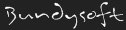L3DT documentationLarge 3D terrain generator

Water-mapping algorithms

Flooding sea and lakes

I still haven't written a description of water flooding here, but nobody has asked. The basic concept is relatively easy, but the optimised implementation in L3DT is fairly scary.

Water table and salinity mapping

The water table modelling algorithm in L3DT calculates a water 'heightfield' that roughly follows the rise and fall of the terrain whilst smoothly connecting all of the water bodies (sea, lakes, etc). The purpose of calculating the water table is to allow the attributes map algorithm to assign water-dependent land types such as lake mud, beach sand, lush grass and deep desert.

In outline, the algorithm calculates the height of the water table at each pixel using the terrain and water table height of its neighbours, and then iterates several times to reach a stable solution. In algebraic form, a change in the terrain height (δh) when moving from point a to point b causes a corresponding change in the height of the water table (δw) that may be expressed as:

δwab = cgrad × δhab - closs

where:

• cgrad is an arbitrary coefficient of the gradient1), and;
• closs is an arbitrary linear loss coefficient.

Using the above equation, the algorithm rasters across the map in various directions2) calculating the height of the water table at each pixel, based on the water table height of the last pixel and the change in terrain height from the last pixel to the current pixel. If the calculated value is greater than the current water table height, the water table is set to the new height and the algorithm moves on to another pixel. The procedure is iterated a few times to achieve a satisfactory solution.

This equation is a massive simplification of genuine hydrological-modelling, with all of the complex parameters such as soil porosity, rainfall, and so-forth being hidden in those coefficients. They are, therefore, effectively arbitrary, so please feel-free to tinker with them in the 'water table modelling' wizard.

Salinity is handled in a similar manner to the water-table height, but with a slightly different equation that has, at best, marginal grounding in reality.Combined water body, water-table and salinity display.

There are more pretty screenshots of this in the water map display schemes guide.

Rivers

The RainMaker plugin is about all I've done for rivers so far. I intend to revisit this someday.

1) In my implementation, the gradient coefficient is split into two (crise and cfall) for rising or falling land.
2) Eight raster orders are used for this.

l3dt/algorithms/wm.txt · Last modified: 2017/08/31 05:35 (external edit)